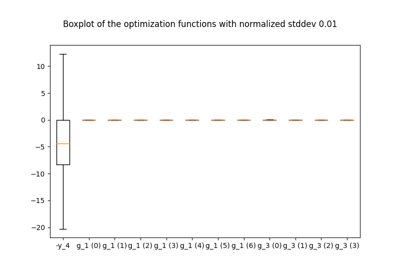gemseo / post

# robustness module¶

Boxplots to quantify the robustness of the optimum.

class gemseo.post.robustness.Robustness(opt_problem)[source]

Uncertainty quantification at the optimum.

Compute the quadratic approximations of all the output functions, propagate analytically a normal distribution centered on the optimal design variables with a standard deviation which is a percentage of the mean passed in option (default: 1%) and plot the corresponding output boxplot.

Parameters:

opt_problem (OptimizationProblem) – The optimization problem to be post-processed.

Raises:

ValueError – If the JSON grammar file for the options of the post-processor does not exist.

check_options(**options)

Check the options of the post-processor.

Parameters:

**options (Union[int, float, str, bool, Sequence[str]]) – The options of the post-processor.

Raises:

InvalidDataException – If an option is invalid according to the grammar.

Return type:

None

execute(save=True, show=False, file_path=None, directory_path=None, file_name=None, file_extension=None, fig_size=None, **options)

Post-process the optimization problem.

Parameters:
• save (bool) –

If True, save the figure.

By default it is set to True.

• show (bool) –

If True, display the figure.

By default it is set to False.

• file_path (str | Path | None) – The path of the file to save the figures. If the extension is missing, use file_extension. If None, create a file path from directory_path, file_name and file_extension.

• directory_path (str | Path | None) – The path of the directory to save the figures. If None, use the current working directory.

• file_name (str | None) – The name of the file to save the figures. If None, use a default one generated by the post-processing.

• file_extension (str | None) – A file extension, e.g. ‘png’, ‘pdf’, ‘svg’, … If None, use a default file extension.

• fig_size (tuple[float, float] | None) – The width and height of the figure in inches, e.g. (w, h). If None, use the OptPostProcessor.DEFAULT_FIG_SIZE of the post-processor.

• **options (OptPostProcessorOptionType) – The options of the post-processor.

Returns:

The figures, to be customized if not closed.

Raises:

ValueError – If the opt_problem.database is empty.

DEFAULT_FIG_SIZE = (8.0, 5.0)

The default width and height of the figure, in inches.

Type:
SR1_APPROX = 'SR1'
database: Database

The database generated by the optimization problem.

property figures: dict[str, matplotlib.figure.Figure]

The Matplotlib figures indexed by a name, or the nameless figure counter.

materials_for_plotting: dict[Any, Any]

The materials to eventually rebuild the plot in another framework.

opt_problem: OptimizationProblem

The optimization problem.

property output_files: list[str]

The paths to the output files.

gemseo.post.robustness.normal(loc=0.0, scale=1.0, size=None)

Draw random samples from a normal (Gaussian) distribution.

The probability density function of the normal distribution, first derived by De Moivre and 200 years later by both Gauss and Laplace independently , is often called the bell curve because of its characteristic shape (see the example below).

The normal distributions occurs often in nature. For example, it describes the commonly occurring distribution of samples influenced by a large number of tiny, random disturbances, each with its own unique distribution .

Note

New code should use the normal method of a default_rng() instance instead; please see the Quick Start.

Parameters:
• loc (float or array_like of floats) – Mean (“centre”) of the distribution.

• scale (float or array_like of floats) – Standard deviation (spread or “width”) of the distribution. Must be non-negative.

• size (int or tuple of ints, optional) – Output shape. If the given shape is, e.g., (m, n, k), then m * n * k samples are drawn. If size is None (default), a single value is returned if loc and scale are both scalars. Otherwise, np.broadcast(loc, scale).size samples are drawn.

Returns:

out – Drawn samples from the parameterized normal distribution.

Return type:

ndarray or scalar

scipy.stats.norm

probability density function, distribution or cumulative density function, etc.

random.Generator.normal

which should be used for new code.

Notes

The probability density for the Gaussian distribution is

$p(x) = \frac{1}{\sqrt{ 2 \pi \sigma^2 }} e^{ - \frac{ (x - \mu)^2 } {2 \sigma^2} },$

where $$\mu$$ is the mean and $$\sigma$$ the standard deviation. The square of the standard deviation, $$\sigma^2$$, is called the variance.

The function has its peak at the mean, and its “spread” increases with the standard deviation (the function reaches 0.607 times its maximum at $$x + \sigma$$ and $$x - \sigma$$ ). This implies that normal is more likely to return samples lying close to the mean, rather than those far away.

References

Examples

Draw samples from the distribution:

>>> mu, sigma = 0, 0.1 # mean and standard deviation
>>> s = np.random.normal(mu, sigma, 1000)


Verify the mean and the variance:

>>> abs(mu - np.mean(s))
0.0  # may vary

>>> abs(sigma - np.std(s, ddof=1))
0.1  # may vary


Display the histogram of the samples, along with the probability density function:

>>> import matplotlib.pyplot as plt
>>> count, bins, ignored = plt.hist(s, 30, density=True)
>>> plt.plot(bins, 1/(sigma * np.sqrt(2 * np.pi)) *
...                np.exp( - (bins - mu)**2 / (2 * sigma**2) ),
...          linewidth=2, color='r')
>>> plt.show()


Two-by-four array of samples from N(3, 6.25):

>>> np.random.normal(3, 2.5, size=(2, 4))
array([[-4.49401501,  4.00950034, -1.81814867,  7.29718677],   # random
[ 0.39924804,  4.68456316,  4.99394529,  4.84057254]])  # random

gemseo.post.robustness.seed(self, seed=None)

Reseed a legacy MT19937 BitGenerator

Notes

This is a convenience, legacy function.

The best practice is to not reseed a BitGenerator, rather to recreate a new one. This method is here for legacy reasons. This example demonstrates best practice.

>>> from numpy.random import MT19937
>>> from numpy.random import RandomState, SeedSequence
>>> rs = RandomState(MT19937(SeedSequence(123456789)))
# Later, you want to restart the stream
>>> rs = RandomState(MT19937(SeedSequence(987654321)))


## Examples using Robustness¶Robustness

Robustness